# ETAA Math Midterm

You have 50 minutes to complete this assessment.

Please Note: Do not do the last section, we haven’t studied that math item yet.

For Questions #1-#4, find the numeric solution to each question.

#### Numerical Sequences

For Questions #5-#16, find the missing number in each numeric sequence.

#### Plug In PEMDAS

For Questions #17-#18, substitute the numbers for the variables, simplify, and find the numeric solution.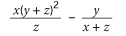For Questions #19-#20, substitute the numbers for the variables, simplify, and find the numeric solution.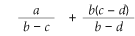For Questions #21-#22, substitute the numbers for the variables, simplify, and find the numeric solution.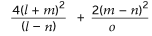For Questions #23-#24, substitute the numbers for the variables, simplify, and find the numeric solution.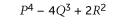For Questions #25-#26, substitute the numbers for the variables, simplify, and find the numeric solution.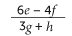For Questions #27-#28, substitute the numbers for the variables, simplify, and find the numeric solution.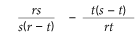#### Linear Equations

For Questions #29-#34, Match the Linear Equation expression in the question to the identical linear equation in the answers.
PLEASE IGNORE THE ROMAN NUMERALS ABOVE THE GRAPHS

29.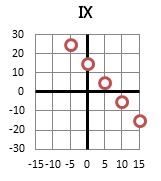A. sixteen more than twice a number
B. y = -3x + 15
C.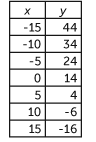D. fourteen more than half the opposite of a number
ABCD

30.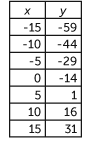A. Five more than a number
B. y = –3x – 14
C. Fourteen less than three times a number
D.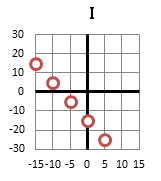ABCD

31. y = –4x + 8

A.B. Four less than eight times a number
C.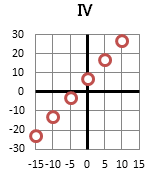D. Eight more than four times a number
ABCD

32. Five less than twice a number

A.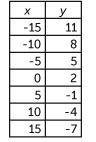B. y = 2x – 5
C.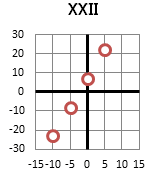D. y = –5x + 2
ABCD

33.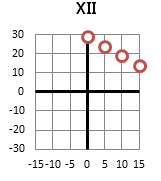A. y = 2x + 5
B.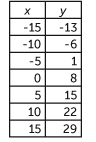C. y = –x + 30
D. Six more than three times a number
ABCD

34. Two more than four times a number

A. y = 2x + 4
B.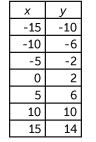C.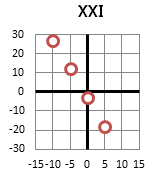D.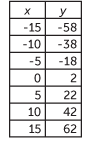ABCD

#### Inverse Functions

Each equation in Questions #35-#38 has y all by itself on the left side of the equal sign. Applying algebra rules, move everything to the left side of the equals sign, except the x variable.